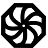# Programming-IdiomsImplementation
C#

Be concise.

Be useful.

All contributions dictatorially edited by webmasters to match personal tastes.

Please try to avoid dependencies to third-party libraries and frameworks.

Other implementations
`import "math/big"`
```nb := big.NewInt(int64(n))
var z big.Int
z.Exp(x, nb, nil)```
`import java.math.BigInteger;`
`BigInteger z = x.pow(n);`
`use bigint;`
```my (\$x, \$y) = (3 ** 200, 25);
my \$z = \$x ** \$y;```
`import std.bigint;`
`BigInt z = x ^^ n;`
`z = x**n`
`extern crate num;`
`let z = num::pow(x, n);`
`import "dart:math";`
`var z = pow(x, n);`
`z = x ** n`
`z = :math.pow(x, n)`
`z = x ^ n`
`let z = x**n`